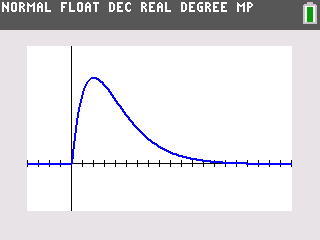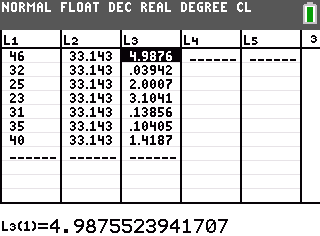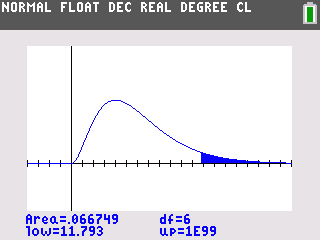••• ##### Device
• TI-84 Plus
• TI-84 Plus Silver Edition
•TI-84 Plus C Silver Edition
•TI-84 Plus CE
• ##### Report an Issue

Statistics: Goodness-Of-Fit
by Texas Instruments#### Overview

Students test claims of whether given distributions "fit" theoretical distributions.

#### Key Steps

•In Problem 1, students are introduced to the goodness-of-fit test and the test statistic. They also observe a graph and some may recognize the positively skewed chi square distribution. They can change the degrees of freedom and determine what large and small chi-square values would indicate.

•Students investigate a scenario presented that tests a claim that every category has the same proportion. They will use the List Editor and the sum command to calculate the chi-square value and a Chi-Square distribution chart to find the critical value.

•Finally, students will use the Shade command to calculate the P-value. At the end of this activity, students will be able to use a chi-squared test to test the hypothesis that an observed frequency distribution fits an expected frequency distribution.